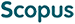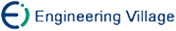吴 刚. 隐含多项式曲线曲面拟合次数的确定研究[J]. 计算机研究与发展, 2007, 44(1): 148-153.
 引用本文: 吴 刚. 隐含多项式曲线曲面拟合次数的确定研究[J]. 计算机研究与发展, 2007, 44(1): 148-153.Wu Gang. Research on Degree of Fitting Implicit Polynomial Curves and Surfaces[J]. Journal of Computer Research and Development, 2007, 44(1): 148-153.
 Citation: Wu Gang. Research on Degree of Fitting Implicit Polynomial Curves and Surfaces[J]. Journal of Computer Research and Development, 2007, 44(1): 148-153.## Research on Degree of Fitting Implicit Polynomial Curves and Surfaces

• 摘要: 选用合适次数的隐含多项式曲线曲面描述目标物体是处理和识别目标物体的关键，因而需要在理论上解决隐含多项式曲线或者曲面的次数确定问题.根据目标物体本身的特征，从理论上得出隐含多项式曲线描述物体的次数确定定理，并给出了具体计算公式.该方法首先由给定物体边界的轮廓检测出其驻点数，然后根据驻点数得到拟合隐含多项式曲线方程次数的下界，进而推广到三维物体的隐含多项式曲面拟合次数的确定.最后给出的应用实例进一步验证了算法的有效性与可操作性.

Abstract: How to choose suitable degree of fitting implicit polynomial curves and surfaces is a key problem when the objects are described and recognized by implicit polynomials, but the problem is not solved in theory. A theorem is presented to determine the degree of fitting implicit polynomials based on the object boundary features, and the formula for the degree of fitting implicit polynomial is given. From the theory, the algorithm for determination of the degree of implicit polynomials is followed: detecting the stationary points in the object boundary based on its features, and then calculating the minimum degree according to the formula derived from the theorem. In addition, the theory can be extended to three-dimensional object. Finally, experiments are given to illustrate the efficiency and operation of the algorithm./下载:  全尺寸图片 幻灯片
• 分享
• 用微信扫码二维码

分享至好友和朋友圈